# Transformer代码完全解读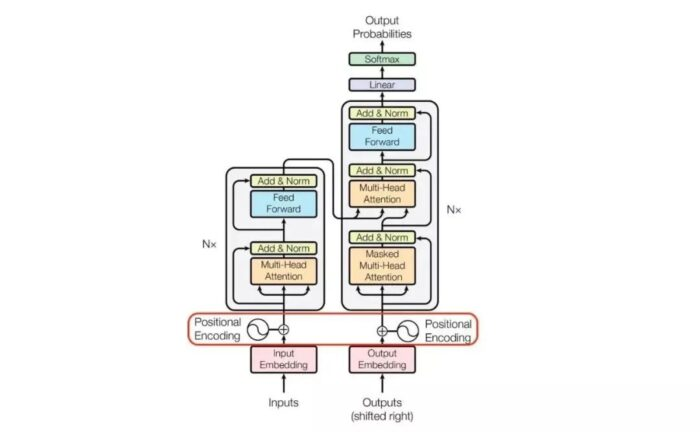2017年谷歌在一篇名为《Attention Is All  You  Need》的论文中,提出了一个基于attention(自注意力机制)结构来处理序列相关的问题的模型，名为Transformer。

Transformer在很多不同nlp任务中获得了成功，例如：文本分类、机器翻译、阅读理解等。在解决这类问题时，Transformer模型摒弃了固有的定式，并没有用任何CNN或者RNN的结构，而是使用了Attention注意力机制，自动捕捉输入序列不同位置处的相对关联，善于处理较长文本，并且该模型可以高度并行地工作，训练速度很快。

## 1 模型结构概览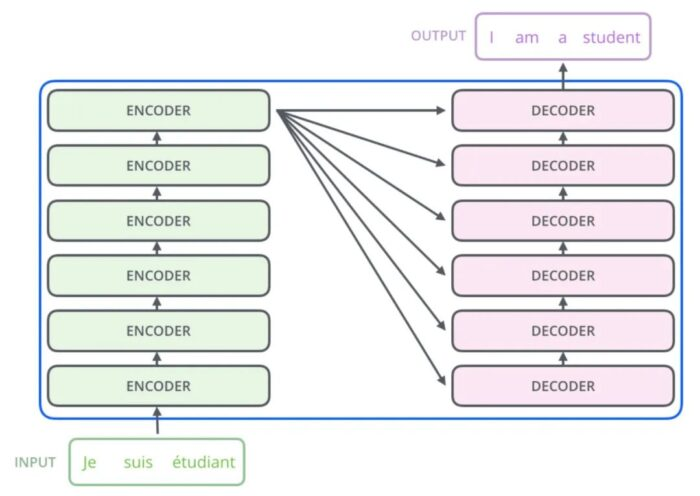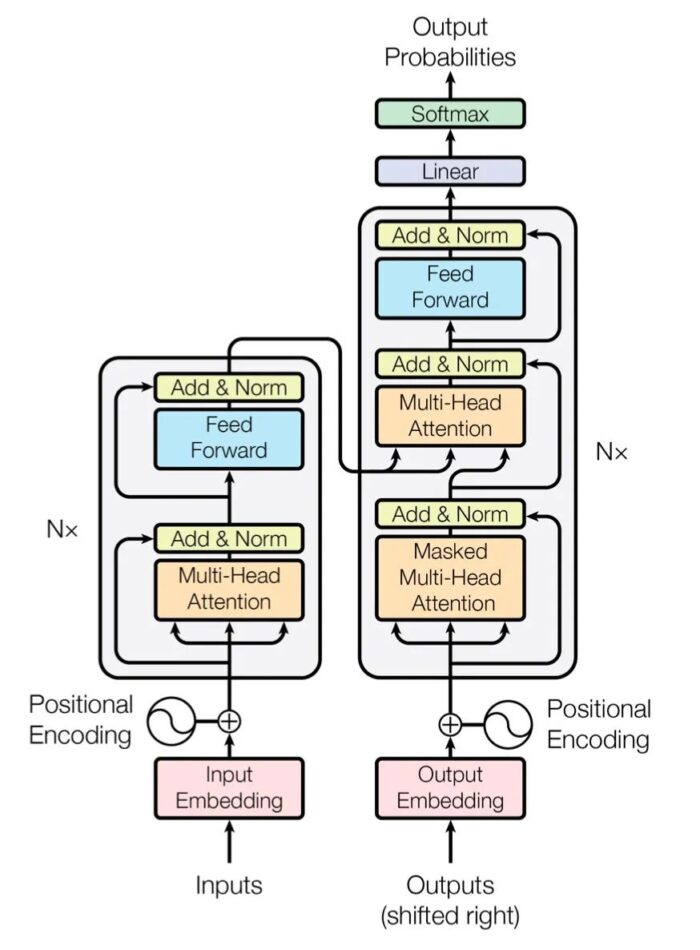## 2 模型输入

### 2.1 Embedding层

Embedding层的作用是将某种格式的输入数据，例如文本，转变为模型可以处理的向量表示，来描述原始数据所包含的信息。

`Embedding`层输出的可以理解为当前时间步的特征，如果是文本任务，这里就可以是`Word Embedding`，如果是其他任务，就可以是任何合理方法所提取的特征。

```class Embeddings(nn.Module):
def __init__(self, d_model, vocab):
"""
类的初始化函数
d_model：指词嵌入的维度
vocab:指词表的大小
"""
super(Embeddings, self).__init__()
#之后就是调用nn中的预定义层Embedding，获得一个词嵌入对象self.lut
self.lut = nn.Embedding(vocab, d_model)
#最后就是将d_model传入类中
self.d_model =d_model
def forward(self, x):
"""
Embedding层的前向传播逻辑
参数x：这里代表输入给模型的单词文本通过词表映射后的one-hot向量
将x传给self.lut并与根号下self.d_model相乘作为结果返回
"""
embedds = self.lut(x)
return embedds * math.sqrt(self.d_model)```

### 2.2 位置编码：

`Positional Encodding`位置编码的作用是为模型提供当前时间步的前后出现顺序的信息。因为Transformer不像RNN那样的循环结构有前后不同时间步输入间天然的先后顺序，所有的时间步是同时输入，并行推理的，因此在时间步的特征中融合进位置编码的信息是合理的。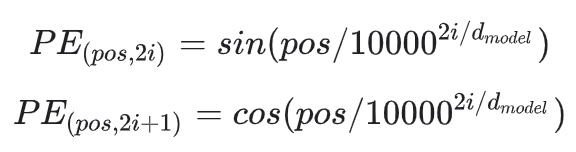```class PositionalEncoding(nn.Module):
def __init__(self, d_model, dropout, max_len=5000):
"""
位置编码器类的初始化函数

共有三个参数，分别是
d_model：词嵌入维度
dropout: dropout触发比率
max_len：每个句子的最大长度
"""
super(PositionalEncoding, self).__init__()
self.dropout = nn.Dropout(p=dropout)

# Compute the positional encodings
# 注意下面代码的计算方式与公式中给出的是不同的，但是是等价的，你可以尝试简单推导证明一下。
# 这样计算是为了避免中间的数值计算结果超出float的范围，
pe = torch.zeros(max_len, d_model)
position = torch.arange(0, max_len).unsqueeze(1)
div_term = torch.exp(torch.arange(0, d_model, 2) *
-(math.log(10000.0) / d_model))
pe[:, 0::2] = torch.sin(position * div_term)
pe[:, 1::2] = torch.cos(position * div_term)
pe = pe.unsqueeze(0)
self.register_buffer( pe , pe)

def forward(self, x):
x = x   Variable(self.pe[:, :x.size(1)], requires_grad=False)
return self.dropout(x)```

### 2.3 Encoder和Decoder都包含输入模块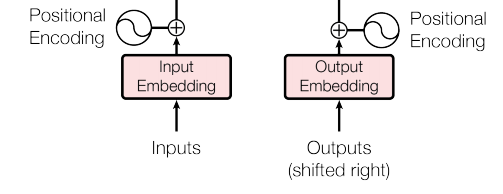## 3 Encoder

### 3.1 编码器

```# 定义一个clones函数，来更方便的将某个结构复制若干份
def clones(module, N):
"Produce N identical layers."
return nn.ModuleList([copy.deepcopy(module) for _ in range(N)])

class Encoder(nn.Module):
"""
Encoder
The encoder is composed of a stack of N=6 identical layers.
"""
def __init__(self, layer, N):
super(Encoder, self).__init__()
# 调用时会将编码器层传进来，我们简单克隆N分，叠加在一起，组成完整的Encoder
self.layers = clones(layer, N)
self.norm = LayerNorm(layer.size)

def forward(self, x, mask):
"Pass the input (and mask) through each layer in turn."
for layer in self.layers:
x = layer(x, mask)
return self.norm(x)```

### 3.2 编码器层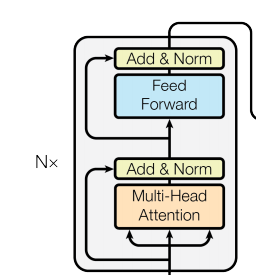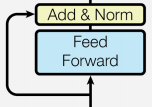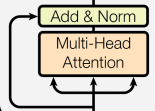```class SublayerConnection(nn.Module):
"""
实现子层连接结构的类
"""
def __init__(self, size, dropout):
super(SublayerConnection, self).__init__()
self.norm = LayerNorm(size)
self.dropout = nn.Dropout(dropout)

def forward(self, x, sublayer):

# 原paper的方案
#sublayer_out = sublayer(x)
#x_norm = self.norm(x   self.dropout(sublayer_out))

# 稍加调整的版本
sublayer_out = sublayer(x)
sublayer_out = self.dropout(sublayer_out)
x_norm = x   self.norm(sublayer_out)
return x_norm```

```class EncoderLayer(nn.Module):
"EncoderLayer is made up of two sublayer: self-attn and feed forward"
def __init__(self, size, self_attn, feed_forward, dropout):
super(EncoderLayer, self).__init__()
self.self_attn = self_attn
self.feed_forward = feed_forward
self.sublayer = clones(SublayerConnection(size, dropout), 2)
self.size = size   # embedding s dimention of model, 默认512

def forward(self, x, mask):
# attention sub layer
x = self.sublayer(x, lambda x: self.self_attn(x, x, x, mask))
# feed forward sub layer
z = self.sublayer(x, self.feed_forward)
return z```

### 3.3 注意力机制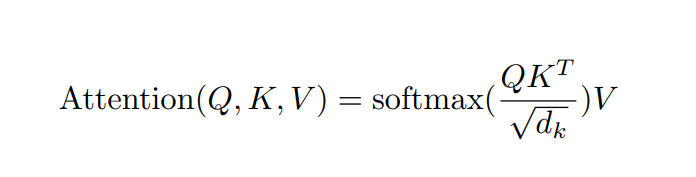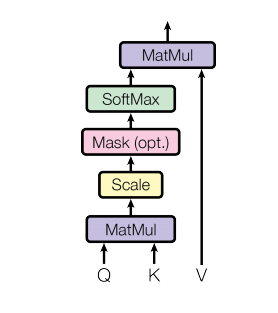DataWhale开源项目：https://github.com/datawhalechina/learn-nlp-with-transformer

```def attention(query, key, value, mask=None, dropout=None):
"Compute  Scaled Dot Product Attention "

#首先取query的最后一维的大小，对应词嵌入维度
d_k = query.size(-1)
#按照注意力公式，将query与key的转置相乘，这里面key是将最后两个维度进行转置，再除以缩放系数得到注意力得分张量scores
scores = torch.matmul(query, key.transpose(-2, -1)) / math.sqrt(d_k)

#接着判断是否使用掩码张量
if mask is not None:

#对scores的最后一维进行softmax操作，使用F.softmax方法，这样获得最终的注意力张量
p_attn = F.softmax(scores, dim = -1)

#之后判断是否使用dropout进行随机置0
if dropout is not None:
p_attn = dropout(p_attn)

#最后，根据公式将p_attn与value张量相乘获得最终的query注意力表示，同时返回注意力张量

### 3.4 多头注意力机制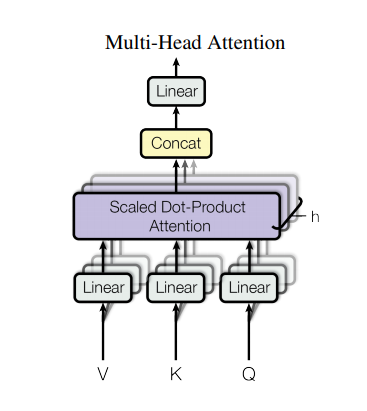```class MultiHeadedAttention(nn.Module):
def __init__(self, h, d_model, dropout=0.1):
#在类的初始化时，会传入三个参数，h代表头数，d_model代表词嵌入的维度，dropout代表进行dropout操作时置0比率，默认是0.1
assert d_model % h == 0
#得到每个头获得的分割词向量维度d_k
self.d_k = d_model // h
#传入头数h
self.h = h

#创建linear层，通过nn的Linear实例化，它的内部变换矩阵是embedding_dim x embedding_dim，然后使用，为什么是四个呢，这是因为在多头注意力中，Q,K,V各需要一个，最后拼接的矩阵还需要一个，因此一共是四个
self.linears = clones(nn.Linear(d_model, d_model), 4)
#self.attn为None，它代表最后得到的注意力张量，现在还没有结果所以为None
self.attn = None
self.dropout = nn.Dropout(p=dropout)

def forward(self, query, key, value, mask=None):
if mask is not None:
# Same mask applied to all h heads.
#使用unsqueeze扩展维度，代表多头中的第n头
#接着，我们获得一个batch_size的变量，他是query尺寸的第1个数字，代表有多少条样本
nbatches = query.size(0)

# 1) Do all the linear projections in batch from d_model => h x d_k
# 首先利用zip将输入QKV与三个线性层组到一起，然后利用for循环，将输入QKV分别传到线性层中，做完线性变换后，开始为每个头分割输入，这里使用view方法对线性变换的结构进行维度重塑，多加了一个维度h代表头，这样就意味着每个头可以获得一部分词特征组成的句子，其中的-1代表自适应维度，计算机会根据这种变换自动计算这里的值，然后对第二维和第三维进行转置操作，为了让代表句子长度维度和词向量维度能够相邻，这样注意力机制才能找到词义与句子位置的关系，从attention函数中可以看到，利用的是原始输入的倒数第一和第二维，这样我们就得到了每个头的输入
query, key, value =
[l(x).view(nbatches, -1, self.h, self.d_k).transpose(1, 2)
for l, x in zip(self.linears, (query, key, value))]

# 2) Apply attention on all the projected vectors in batch.
x, self.attn = attention(query, key, value, mask=mask,
dropout=self.dropout)

# 3) "Concat" using a view and apply a final linear.
# 通过多头注意力计算后，我们就得到了每个头计算结果组成的4维张量，我们需要将其转换为输入的形状以方便后续的计算，因此这里开始进行第一步处理环节的逆操作，先对第二和第三维进行转置，然后使用contiguous方法。这个方法的作用就是能够让转置后的张量应用view方法，否则将无法直接使用，所以，下一步就是使用view重塑形状，变成和输入形状相同。
x = x.transpose(1, 2).contiguous()
.view(nbatches, -1, self.h * self.d_k)
#最后使用线性层列表中的最后一个线性变换得到最终的多头注意力结构的输出
return self.linears[-1](x)```

### 3.5 前馈全连接层

EncoderLayer中另一个核心的子层是 Feed Forward Layer，我们这就介绍一下。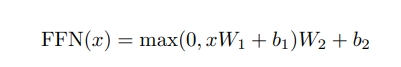Feed Forward Layer 其实就是简单的由两个前向全连接层组成，核心在于，Attention模块每个时间步的输出都整合了所有时间步的信息，而Feed Forward Layer每个时间步只是对自己的特征的一个进一步整合，与其他时间步无关。

```class PositionwiseFeedForward(nn.Module):
def __init__(self, d_model, d_ff, dropout=0.1):
#初始化函数有三个输入参数分别是d_model，d_ff，和dropout=0.1，第一个是线性层的输入维度也是第二个线性层的输出维度，因为我们希望输入通过前馈全连接层后输入和输出的维度不变，第二个参数d_ff就是第二个线性层的输入维度和第一个线性层的输出，最后一个是dropout置0比率。
super(PositionwiseFeedForward, self).__init__()
self.w_1 = nn.Linear(d_model, d_ff)
self.w_2 = nn.Linear(d_ff, d_model)
self.dropout = nn.Dropout(dropout)

def forward(self, x):
#输入参数为x，代表来自上一层的输出，首先经过第一个线性层，然后使用F中的relu函数进行激活，之后再使用dropout进行随机置0，最后通过第二个线性层w2，返回最终结果
return self.w_2(self.dropout(F.relu(self.w_1(x))))```

### 3.6. 规范化层

Transformer中使用的normalization手段是layer norm，实现代码很简单，如下：

```class LayerNorm(nn.Module):
"Construct a layernorm module (See citation for details)."
def __init__(self, feature_size, eps=1e-6):
#初始化函数有两个参数，一个是features,表示词嵌入的维度,另一个是eps它是一个足够小的数，在规范化公式的分母中出现,防止分母为0，默认是1e-6。
super(LayerNorm, self).__init__()
#根据features的形状初始化两个参数张量a2，和b2，第一初始化为1张量，也就是里面的元素都是1，第二个初始化为0张量，也就是里面的元素都是0，这两个张量就是规范化层的参数。因为直接对上一层得到的结果做规范化公式计算，将改变结果的正常表征，因此就需要有参数作为调节因子，使其即能满足规范化要求，又能不改变针对目标的表征，最后使用nn.parameter封装，代表他们是模型的参数
self.a_2 = nn.Parameter(torch.ones(feature_size))
self.b_2 = nn.Parameter(torch.zeros(feature_size))
#把eps传到类中
self.eps = eps

def forward(self, x):
#输入参数x代表来自上一层的输出，在函数中，首先对输入变量x求其最后一个维度的均值，并保持输出维度与输入维度一致，接着再求最后一个维度的标准差，然后就是根据规范化公式，用x减去均值除以标准差获得规范化的结果。
#最后对结果乘以我们的缩放参数，即a2,*号代表同型点乘，即对应位置进行乘法操作，加上位移参b2，返回即可
mean = x.mean(-1, keepdim=True)
std = x.std(-1, keepdim=True)
return self.a_2 * (x - mean) / (std   self.eps)   self.b_2```

### 3.7 掩码及其作用

```def subsequent_mask(size):
#生成向后遮掩的掩码张量，参数size是掩码张量最后两个维度的大小，它最后两维形成一个方阵

"Mask out subsequent positions."
attn_shape = (1, size, size)

#然后使用np.ones方法向这个形状中添加1元素，形成上三角阵
subsequent_mask = np.triu(np.ones(attn_shape), k=1).astype( uint8 )

## 4 Decoder

### 4.1 解码器整体结构

```#使用类Decoder来实现解码器
class Decoder(nn.Module):
"Generic N layer decoder with masking."
def __init__(self, layer, N):
#初始化函数的参数有两个，第一个就是解码器层layer，第二个是解码器层的个数N
super(Decoder, self).__init__()
#首先使用clones方法克隆了N个layer，然后实例化一个规范化层，因为数据走过了所有的解码器层后最后要做规范化处理。
self.layers = clones(layer, N)
self.norm = LayerNorm(layer.size)

for layer in self.layers:
return self.norm(x)```

### 4.2 解码器层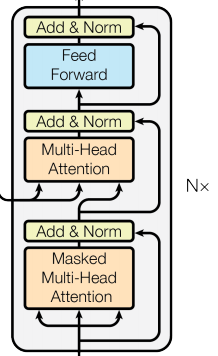```#使用DecoderLayer的类实现解码器层
class DecoderLayer(nn.Module):
"Decoder is made of self-attn, src-attn, and feed forward (defined below)"
def __init__(self, size, self_attn, src_attn, feed_forward, dropout):
#初始化函数的参数有5个，分别是size，代表词嵌入的维度大小，同时也代表解码器的尺寸，第二个是self_attn，多头自注意力对象，也就是说这个注意力机制需要Q=K=V，第三个是src_attn,多头注意力对象，这里Q!=K=V，第四个是前馈全连接层对象，最后就是dropout置0比率
super(DecoderLayer, self).__init__()
self.size = size
self.self_attn = self_attn
self.src_attn = src_attn
self.feed_forward = feed_forward
#按照结构图使用clones函数克隆三个子层连接对象
self.sublayer = clones(SublayerConnection(size, dropout), 3)

#forward函数中的参数有4个，分别是来自上一层的输入x，来自编码器层的语义存储变量memory，以及源数据掩码张量和目标数据掩码张量，将memory表示成m之后方便使用。
"Follow Figure 1 (right) for connections."
m = memory
#将x传入第一个子层结构，第一个子层结构的输入分别是x和self-attn函数，因为是自注意力机制，所以Q,K,V都是x，最后一个参数时目标数据掩码张量，这时要对目标数据进行遮掩，因为此时模型可能还没有生成任何目标数据。
#比如在解码器准备生成第一个字符或词汇时，我们其实已经传入了第一个字符以便计算损失，但是我们不希望在生成第一个字符时模型能利用这个信息，因此我们会将其遮掩，同样生成第二个字符或词汇时，模型只能使用第一个字符或词汇信息，第二个字符以及之后的信息都不允许被模型使用。
x = self.sublayer(x, lambda x: self.self_attn(x, x, x, tgt_mask))
x = self.sublayer(x, lambda x: self.src_attn(x, m, m, src_mask))

#最后一个子层就是前馈全连接子层，经过它的处理后就可以返回结果，这就是我们的解码器结构
return self.sublayer(x, self.feed_forward)```

## 5 模型输出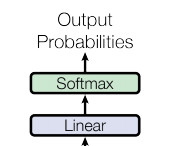```#将线性层和softmax计算层一起实现，因为二者的共同目标是生成最后的结构
#因此把类的名字叫做Generator，生成器类
class Generator(nn.Module):
"Define standard linear   softmax generation step."
def __init__(self, d_model, vocab):
#初始化函数的输入参数有两个，d_model代表词嵌入维度，vocab.size代表词表大小
super(Generator, self).__init__()
#首先就是使用nn中的预定义线性层进行实例化，得到一个对象self.proj等待使用
#这个线性层的参数有两个，就是初始化函数传进来的两个参数：d_model，vocab_size
self.proj = nn.Linear(d_model, vocab)

def forward(self, x):
#前向逻辑函数中输入是上一层的输出张量x,在函数中，首先使用上一步得到的self.proj对x进行线性变化,然后使用F中已经实现的log_softmax进行softmax处理。
return F.log_softmax(self.proj(x), dim=-1)```

## 6 模型构建```# Model Architecture
#使用EncoderDecoder类来实现编码器-解码器结构
class EncoderDecoder(nn.Module):
"""
A standard Encoder-Decoder architecture.
Base for this and many other models.
"""
def __init__(self, encoder, decoder, src_embed, tgt_embed, generator):
#初始化函数中有5个参数，分别是编码器对象，解码器对象,源数据嵌入函数，目标数据嵌入函数，以及输出部分的类别生成器对象.
super(EncoderDecoder, self).__init__()
self.encoder = encoder
self.decoder = decoder
self.src_embed = src_embed    # input embedding module(input embedding   positional encode)
self.tgt_embed = tgt_embed    # ouput embedding module
self.generator = generator    # output generation module

"Take in and process masked src and target sequences."
memory = self.encode(src, src_mask)
return res

def encode(self, src, src_mask):
src_embedds = self.src_embed(src)

target_embedds = self.tgt_embed(tgt)

# Full Model
def make_model(src_vocab, tgt_vocab, N=6, d_model=512, d_ff=2048, h=8, dropout=0.1):
"""
构建模型
params:
src_vocab:
tgt_vocab:
N: 编码器和解码器堆叠基础模块的个数
d_model: 模型中embedding的size，默认512
d_ff: FeedForward Layer层中embedding的size，默认2048
dropout:
"""
c = copy.deepcopy
attn = MultiHeadedAttention(h, d_model)
ff = PositionwiseFeedForward(d_model, d_ff, dropout)
position = PositionalEncoding(d_model, dropout)
model = EncoderDecoder(
Encoder(EncoderLayer(d_model, c(attn), c(ff), dropout), N),
Decoder(DecoderLayer(d_model, c(attn), c(attn), c(ff), dropout), N),
nn.Sequential(Embeddings(d_model, src_vocab), c(position)),
nn.Sequential(Embeddings(d_model, tgt_vocab), c(position)),
Generator(d_model, tgt_vocab))

# This was important from their code.
# Initialize parameters with Glorot / fan_avg.
for p in model.parameters():
if p.dim() > 1:
nn.init.xavier_uniform_(p)
return model```

## 7 实战案例

```# Train the simple copy task.
device = "cuda"
nrof_epochs = 20
batch_size = 32
V = 11    # 词典的数量
sequence_len = 15  # 生成的序列数据的长度
nrof_batch_train_epoch = 30    # 训练时每个epoch多少个batch
nrof_batch_valid_epoch = 10    # 验证时每个epoch多少个batch
criterion = LabelSmoothing(size=V, padding_idx=0, smoothing=0.0)
model = make_model(V, V, N=2)
optimizer = torch.optim.Adam(model.parameters(), lr=0, betas=(0.9, 0.98), eps=1e-9)
model_opt = NoamOpt(model.src_embed.d_model, 1, 400, optimizer)
if device == "cuda":
model.cuda()

for epoch in range(nrof_epochs):
print(f"
epoch {epoch}")
print("train...")
model.train()
data_iter = data_gen(V, sequence_len, batch_size, nrof_batch_train_epoch, device)
loss_compute = SimpleLossCompute(model.generator, criterion, model_opt)
train_mean_loss = run_epoch(data_iter, model, loss_compute, device)
print("valid...")
model.eval()
valid_data_iter = data_gen(V, sequence_len, batch_size, nrof_batch_valid_epoch, device)
valid_loss_compute = SimpleLossCompute(model.generator, criterion, None)
valid_mean_loss = run_epoch(valid_data_iter, model, valid_loss_compute, device)
print(f"valid loss: {valid_mean_loss}")```

```# greedy decode
def greedy_decode(model, src, src_mask, max_len, start_symbol):
memory = model.encode(src, src_mask)
# ys代表目前已生成的序列，最初为仅包含一个起始符的序列，不断将预测结果追加到序列最后
ys = torch.ones(1, 1).fill_(start_symbol).type_as(src.data)
for i in range(max_len-1):
out = model.decode(memory, src_mask,
Variable(ys),
prob = model.generator(out[:, -1])
_, next_word = torch.max(prob, dim = 1)
next_word = next_word.data
ys = torch.cat([ys, torch.ones(1, 1).type_as(src.data).fill_(next_word)], dim=1)
return ys

print("greedy decode")
model.eval()
src = Variable(torch.LongTensor([[1,2,3,4,5,6,7,8,9,10]])).cuda()
src_mask = Variable(torch.ones(1, 1, 10)).cuda()
pred_result = greedy_decode(model, src, src_mask, max_len=10, start_symbol=1)
print(pred_result[:, 1:])```

```...

epoch 18
train...
Epoch Step: 1 Loss: 0.078836 Tokens per Sec: 13734.076172
valid...
Epoch Step: 1 Loss: 0.029015 Tokens per Sec: 23311.662109
valid loss: 0.03555255010724068

epoch 19
train...
Epoch Step: 1 Loss: 0.042386 Tokens per Sec: 13782.227539
valid...
Epoch Step: 1 Loss: 0.022001 Tokens per Sec: 23307.326172
valid loss: 0.014436692930758

greedy decode
tensor([[ 2,  3,  4,  5,  6,  7,  8,  9, 10]], device= cuda:0 )```